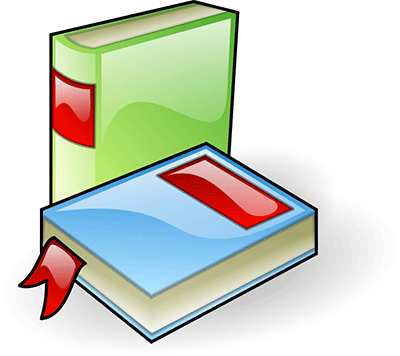ABC-Word.com
We will find the definition for any word

Defenition of the word acceleration

• Defenition of the word acceleration

• The amount by which a speed or velocity increases.
• The change of velocity with respect to time (including deceleration and change of direction).
• The act of increasing the speed of an object.
• The state of having an increasing speed.
• increase in velocity
• (physics) a rate of change of velocity
• the act of accelerating; increasing the speed
• an increase in speed; "modern science caused an acceleration of cultural change"
• an increase in rate of change
• (physics) a rate of increase of velocity

Synonyms for the word acceleration

• hastening
• hurrying
• increase in speed
• increase of rate
• increase of velocity
• quickening
• rushing
• speeding up
• speedup
• stepping up

Similar words in the acceleration

• acceleration
• acceleration's
• accelerations

Hyponyms for the word acceleration

• angular acceleration
• centripetal acceleration
• getaway
• pickup
• precipitation

Hypernyms for the word acceleration

• alteration
• change
• fastness
• hastening
• hurrying
• modification
• rate
• speed
• speeding
• swiftness

Antonyms for the word acceleration

• deceleration
• retardation
• slowing

Idioms for the word acceleration

• acceleration of gravity